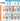## 三方博弈之囚徒困境

Sat, 4th January 2020Edit on Githubgame

# 摘要

2017年10月KeyTo9_Fans先后在知乎数学研发论坛 提问:
3人聚餐后，想玩一个游戏来决定谁买单，这个这个游戏的规则公平吗？

A、B、C 3人约定同时伸出手，每个人都只有手心朝上或者手背朝上2种选择，然后统计手心朝上的人数：
i) 0人或3人则A买单
ii) 1人则B买单
iii) 2人则C买单。

# 问题初探

kastin认为 :
A买单的概率为 $\frac 28$，B买单的概率为 $\frac 38$，C买单的概率为 $\frac 38$，所以A比较占优势。
Fans指出，这只是三方都随机乱出时的结果，但是他们的最优策略不一定是随机乱出。

# 纳什均衡

wayne首先列出了三人的胜率公式:

$P_A(x,y,z) = (1-x)(1-y)(1-z) +xyz$
$P_B(x,y,z) = (1-y)(1-z)x + (1-x)(1-z)y + (1-x)(1-y)z$
$P_C(x,y,z) = (1-z)xy + (1-y)xz + (1-x)yz$

$P_A(y,z) = \frac12(1-y)(1-z) +\frac12yz$
$P_B(y,z) = \frac12(1-z) + \frac12(1-y)z$
$P_C(y,z) = \frac12y + \frac12(1-y)z$

B,C为了能够获得最大利益，那么只要A不使用均匀概率，那么目标就应该让A最大概率买单，所以这时策略就是在A使用不均匀策略时，B和C必须一致的对抗A,但是这会导致B,C两者之间费用严重失衡，正常情况很难维护下去。

# 囚徒困境

KeyTo9_Fans总结知乎上的回复 ，得出:

A必需严格随机出，才可以保证败率不超过$\frac14$。否则A只要有一丁点不随机，就会被BC利用，导致败率大于$\frac12$

（说白了就是BC的策略都是【如果你合作，那么我也合作；如果你背叛，那么我也背叛】并且互相知道，于是在这个前提下，【合作】就变成BC的最佳策略了）

# 串通情况分析

Fans继续给出

（要注意，毁约惩罚的纳什均衡结果是，$A$的败率为$0$$B$$C$的败率为$\frac12$$B$$C$任何一方都不希望这样的结果。）

$A$能否拟定一份新的协议，然后诱惑$B$$C$的其中一方和他签约（比如：保证签约方的败率小于$\frac38$），以获得低于$\frac14$的败率呢？

Github